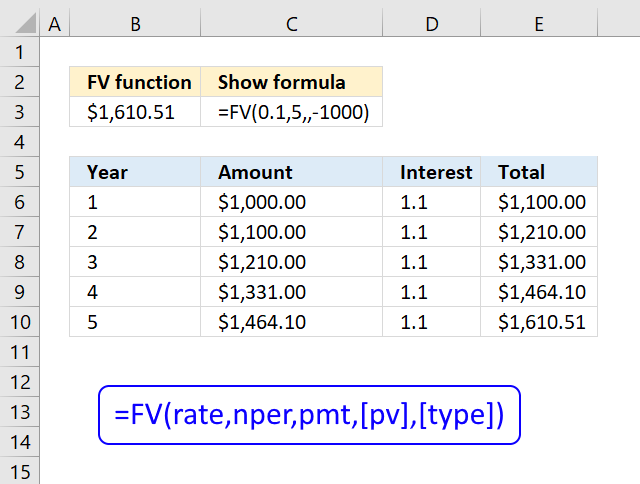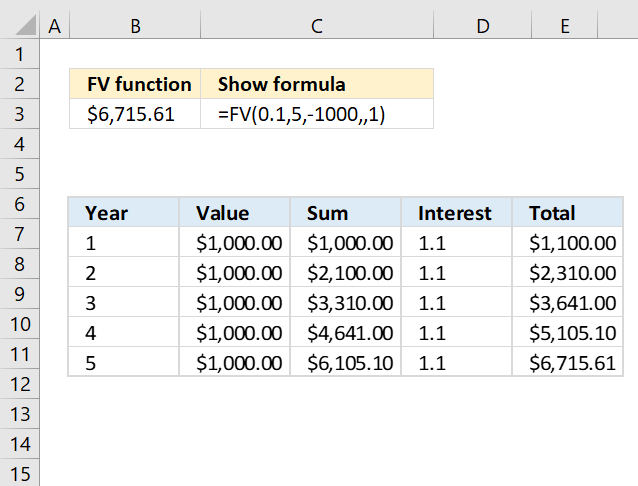Author: Oscar Cronquist Article last updated on May 11, 2022The FV function returns the future value of an investment based on a constant interest rate.

You can use FV with either periodic, constant payments, or a single lump sum payment.

Formula in cell B3:

=FV(0.1,5,,-1000)

The picture above shows a table that calculates the future value of \$1000 with 10% interest rate for 5 years.

### Excel Function Syntax

FV(ratenperpmt, [pv], [type])

### Arguments

 rate Required. The interest rate you want to use. nper Required. The total number of payments. pmt Required. The payment made each period. [pv] Optional. The present value. [type] Optional. When payments are due. 0 (zero) is the default value. 0 - At the end of the period. 1 - At the beginning of the period.

### Example 2The FV function calculates the future value if you save \$1000 each year with an interest rate of 10% for five years.